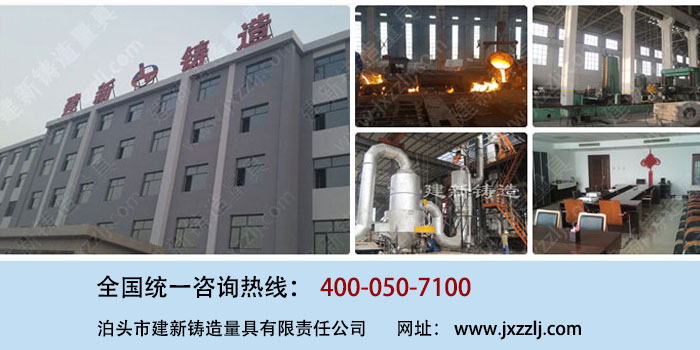# 铸梁平台

## 铸梁平台

1 节约成本：这样不用做成大型的平台,即节省了材料成本,又可以占用很小的空间,可谓是性价比很高。
2 起到支撑的作用
3 减压减震，效果比整体平台好。1500×150×150 1500×200×150 1500×200×200 1500×150×250 2000×150×200 2000×200×200 2000×200×250 2000×250×250 2500×150×200 2500×200×200 2500×200×250 2500×250×250 2750×150×200 2750×200×200 2750×200×250 2750×250×250 3000×150×150 3000×150×200 3000×150×250 3000×200×200 3000×200×250 3000×250×200 3000×250×250 3000×250×300 3500×200×250 3500×200×200 3500×250×250 3500×250×300 4000×200×250 4000×200×200 4000×250×250 4000×250×300 4500×200×250 4500×200×300 4500×250×250 4500×250×300 5000×200×250 5000×200×300 5000×250×250 5000×250×300 特殊规格可根据具体图纸定做

### 地轨地槽系列产品列表